Blog Archives

Working with Date and Time in R

November 2, 2018
By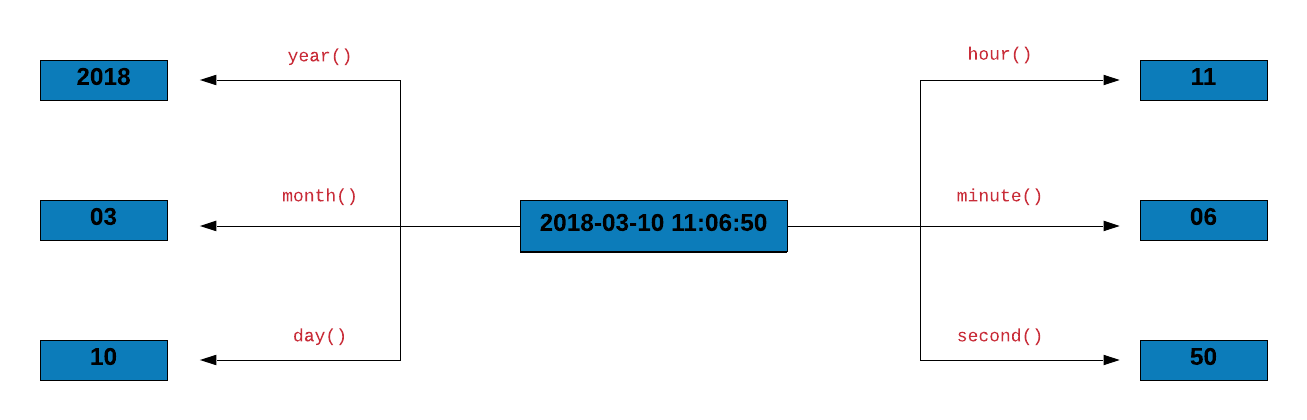Introduction In this post, we will learn to work with date/time data in R using lubridate, an R package that makes it easy to work with dates and time. Let us begin by installing and loading the pacakge. Libraries, Code & Data We will use the following packages: lubridate dplyr magrittr readr The data sets can be downloaded from here and the codes from here. library(lubridate) library(dplyr) library(magrittr) library(readr) Quick Intro Origin Let us look...

Hacking strings with stringr

October 21, 2018
By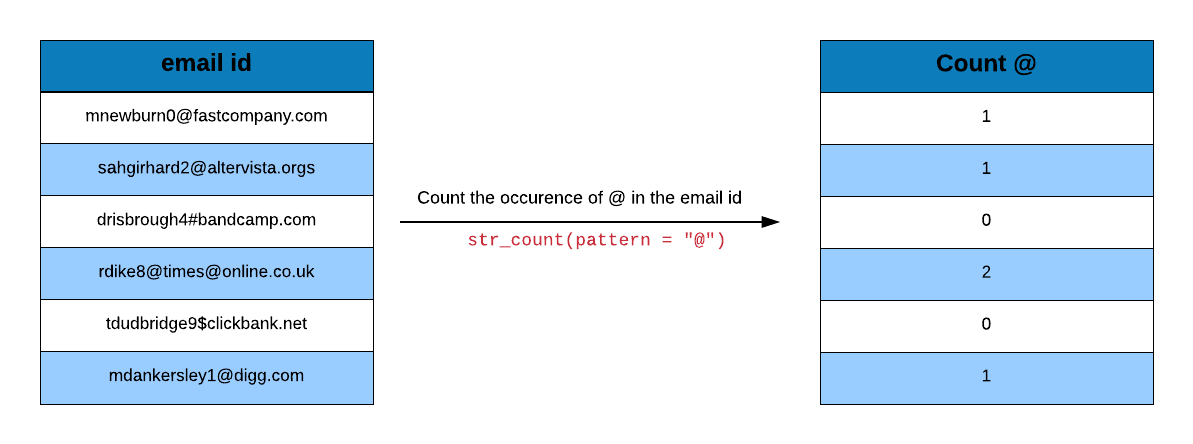Introduction In this post, we will learn to work with string data in R using stringr. As we did in the other posts, we will use a case study to explore the various features of the stringr package. Let us begin by installing and loading stringr and a set of other pacakges we will be using. Libraries, Code & Data We will...

Readable Code with Pipes

October 9, 2018
By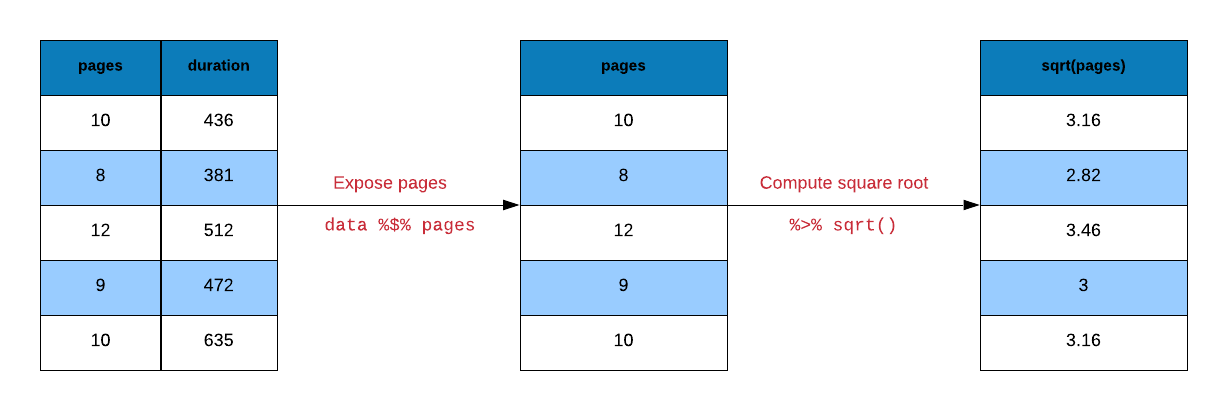Introduction R code contain a lot of parentheses in case of a sequence of multiple operations. When you are dealing with complex code, it results in nested function calls which are hard to read and maintain. The magrittr package by Stefan Milton Bache provides pipes enabling us to write R code that is readable. Pipes allow us to clearly express a sequence...

Introduction to tibbles

September 27, 2018
By

Introduction A tibble, or tbl_df, is a modern reimagining of the data.frame, keeping what time has proven to be effective, and throwing out what is not. Tibbles are data.frames that are lazy and surly: they do less (i.e. they don’t change variable names or types, and don’t do partial matching) and complain more (e.g. when a variable does not exist). This forces...

Data Wrangling with dplyr – Part 3

September 15, 2018
By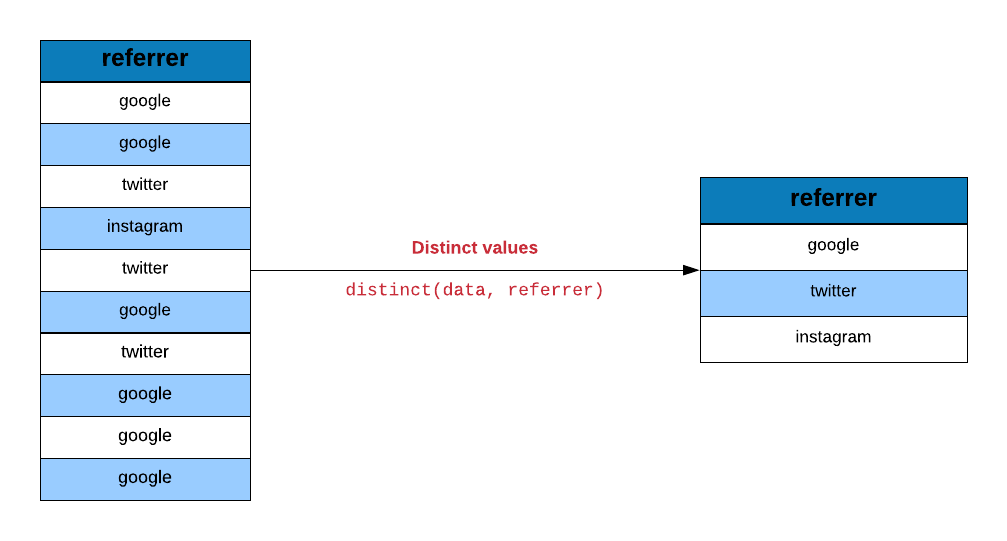Introduction In the previous post, we learnt to combine tables using dplyr. In this post, we will explore a set of helper functions in order to: extract unique rows rename columns sample data extract columns slice rows arrange rows compare tables extract/mutate data using predicate functions count observations for different levels of a variable Libraries, Code & Data We will use the following packages: dplyr readr The data sets can be downloaded from here and the codes...

Data Wrangling with dplyr – Part 2

September 3, 2018
By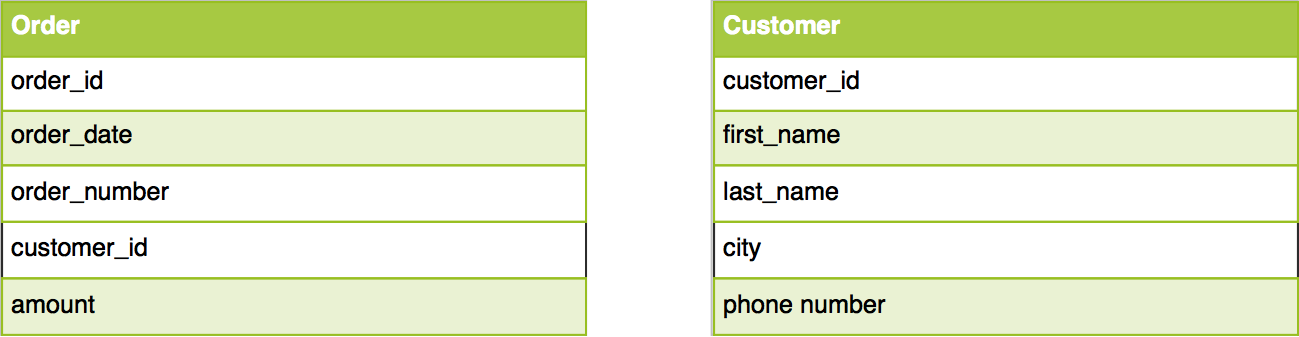Introduction In the previous post we learnt about dplyr verbs and used them to compute average order value for an online retail company data. In this post, we will learn to combine tables using different *_join functions provided in dplyr. Libraries, Code & Data We will use the following packages: dplyr readr The data sets can be downloaded from here and the codes from here. library(dplyr) library(readr) options(tibble.width = Inf) Case Study For...

Data Wrangling with dplyr – Part 1

August 22, 2018
By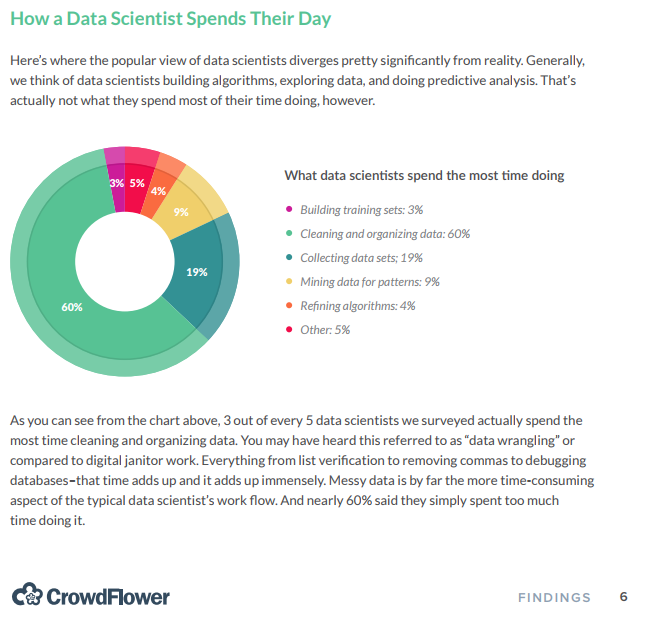Introduction According to a survey by CrowdFlower, data scientists spend most of their time cleaning and manipulating data rather than mining or modeling them for insights. As such, it becomes important to have tools that make data manipulation faster and easier. In today’s post, we introduce you to dplyr, a grammar of data manipulation. Libraries, Code & Data We will use the following libraries: dplyr and readr The data...

Import Data into R – Part 2

August 10, 2018
By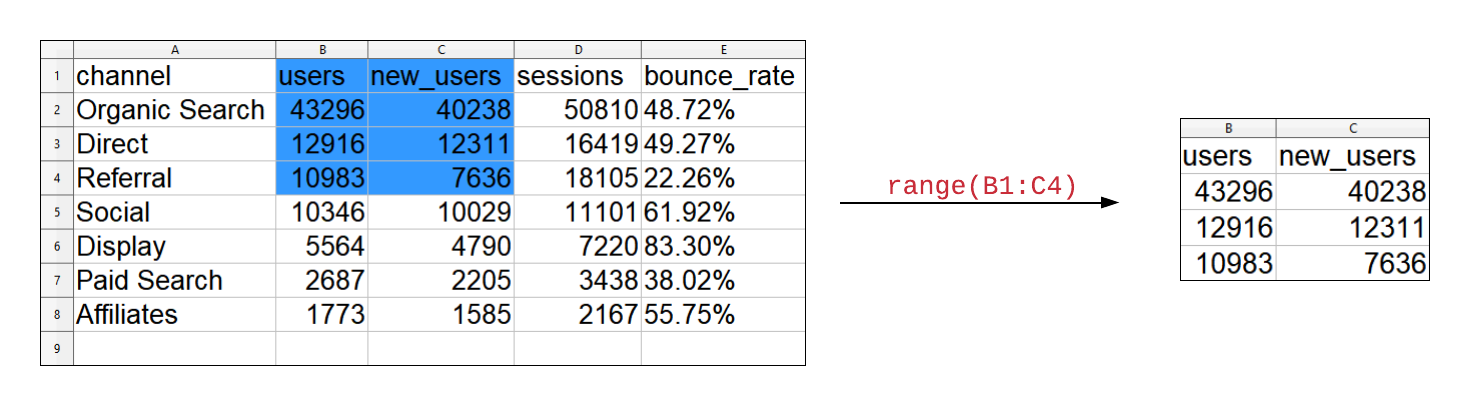Introduction This is the second post in the series Importing Data into R. In the previous post, we explored reading data from flat/delimited files. In this post, we will: list sheets in an excel file read data from an excel sheet read specific cells from an excel sheet read specific rows read specific columns read data from - SAS - SPSS - STATA Libraries, Data & Code We will use the readxl package....

Import Data into R – Part 1

July 29, 2018
By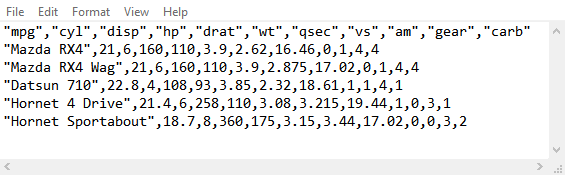Introduction In this post, we will learn to: read data from flat or delimited files handle column names/header skip text/info present before data specify column/variable types read specific columns/variables Libraries, Data & Code We will use the readr package. The data sets can be downloaded from here and the codes from here. library(readr) Types of Delimiters Before we start reading data from files, let us take a quick look at the different types...

ggplot2: Themes

May 6, 2018
By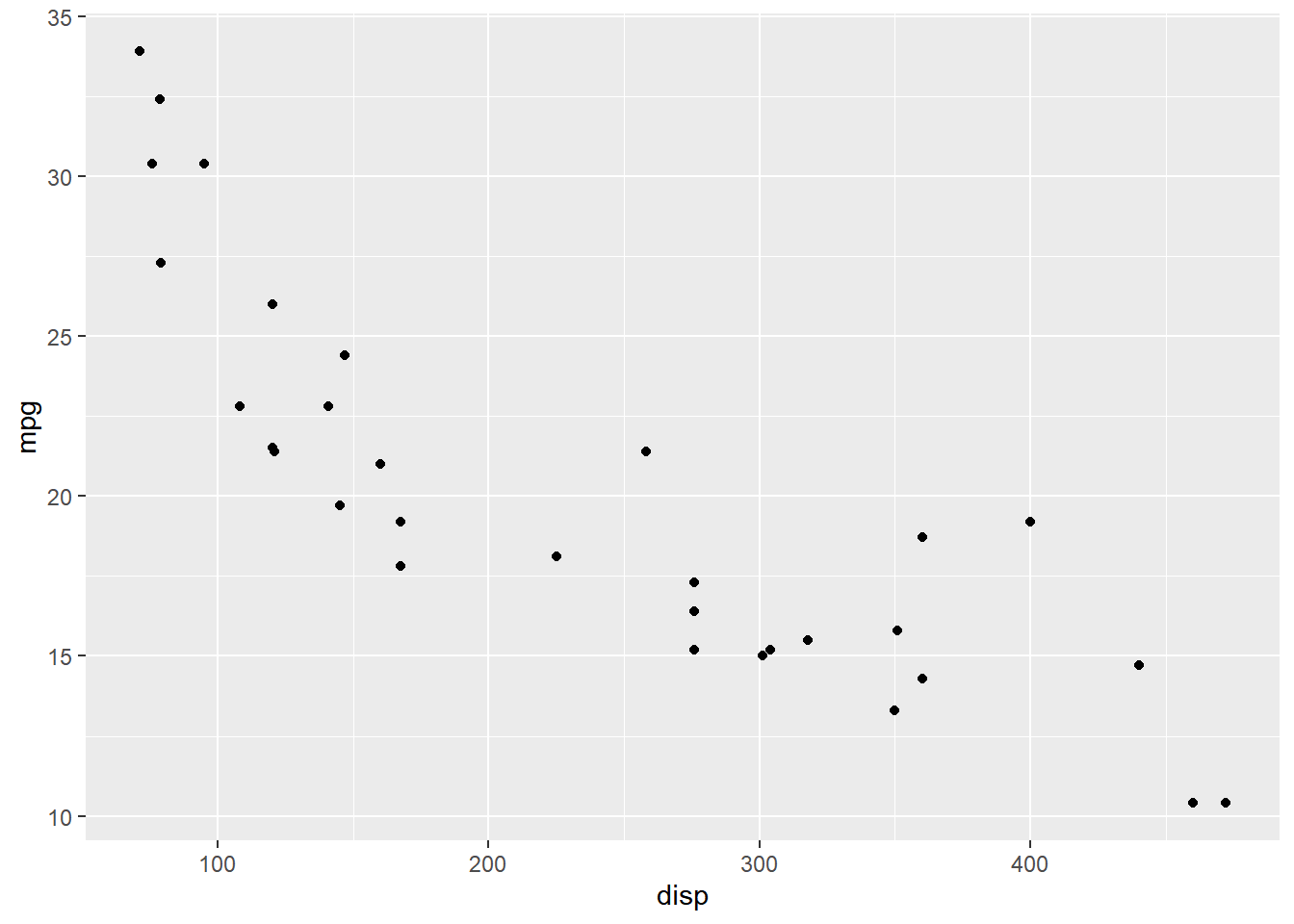Introduction This is the last post in the series Elegant Data Visualization with ggplot2. In the previous post, we learnt to combine multiple plots. In this post, we will learn to modify the appearance of all non data components of the plot such as: axis legend panel plot area background margin facets Libraries, Code & Data We will use the following libraries in this post: readr ggplot2 All the data sets used in this post...